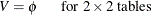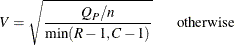#### Chi-Square Tests and Statistics

The CHISQ option provides chi-square tests of homogeneity or independence and measures of association that are based on the chi-square statistic. When you specify the CHISQ option in the TABLES statement, PROC FREQ computes the following chi-square tests for each two-way table: Pearson chi-square, likelihood ratio chi-square, and Mantel-Haenszel chi-square tests. PROC FREQ provides the following measures of association that are based on the Pearson chi-square statistic: phi coefficient, contingency coefficient, and Cramér’s V. Fortables, the CHISQ option also provides Fisher’s exact test and the continuity-adjusted chi-square statistic. You can request Fisher’s exact test for general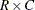tables by specifying the FISHER option in the TABLES or EXACT statement.

If you specify the CHISQ option for one-way tables, PROC FREQ provides a one-way Pearson chi-square goodness-of-fit test. If you specify the CHISQ(LRCHISQ) option for one-way tables, PROC FREQ also provides a one-way likelihood ratio chi-square test. The other tests and statistics that the CHISQ option produces are available only for two-way tables.

For two-way tables, the null hypothesis for the chi-square tests is no association between the row variable and the column variable. When the sample size n is large, the test statistics have asymptotic chi-square distributions under the null hypothesis. When the sample size is not large, or when the data set is sparse or heavily tied, exact tests might be more appropriate than asymptotic tests. PROC FREQ provides exact p-values for the Pearson chi-square, likelihood ratio chi-square, and Mantel-Haenszel chi-square tests, in addition to Fisher’s exact test. For one-way tables, PROC FREQ provides exact p-values for the Pearson and likelihood ratio chi-square goodness-of-fit tests. You can request these exact tests by specifying the corresponding options in the EXACT statement. See the section Exact Statistics for more information.

The Mantel-Haenszel chi-square statistic is appropriate only when both variables lie on an ordinal scale. The other chi-square tests and statistics in this section are appropriate for either nominal or ordinal variables. The following sections give the formulas that PROC FREQ uses to compute the chi-square tests and statistics. For more information about these statistics, see Agresti (2007) and Stokes, Davis, and Koch (2012), and the other references cited.

##### Chi-Square Test for One-Way Tables

For one-way frequency tables, the CHISQ option in the TABLES statement provides a chi-square goodness-of-fit test. Let C denote the number of classes, or levels, in the one-way table. Let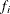denote the frequency of class i (or the number of observations in class i) for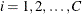. Then PROC FREQ computes the one-way chi-square statistic as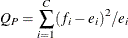where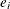is the expected frequency for class i under the null hypothesis.

In the test for equal proportions, which is the default for the CHISQ option, the null hypothesis specifies equal proportions of the total sample size for each class. Under this null hypothesis, the expected frequency for each class equals the total sample size divided by the number of classes,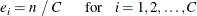In the test for specified frequencies, which PROC FREQ computes when you input null hypothesis frequencies by using the TESTF= option, the expected frequencies are the TESTF= values that you specify. In the test for specified proportions, which PROC FREQ computes when you input null hypothesis proportions by using the TESTP= option, the expected frequencies are determined from the specified TESTP= proportions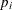as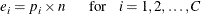Under the null hypothesis (of equal proportions, specified frequencies, or specified proportions),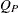has an asymptotic chi-square distribution with C–1 degrees of freedom.

In addition to the asymptotic test, you can request an exact one-way chi-square test by specifying the CHISQ option in the EXACT statement. See the section Exact Statistics for more information.

##### Pearson Chi-Square Test for Two-Way Tables

The Pearson chi-square for two-way tables involves the differences between the observed and expected frequencies, where the expected frequencies are computed under the null hypothesis of independence. The Pearson chi-square statistic is computed as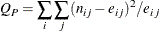where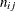is the observed frequency in table cell (i, j) and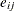is the expected frequency for table cell (i, j). The expected frequency is computed under the null hypothesis that the row and column variables are independent,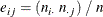When the row and column variables are independent,has an asymptotic chi-square distribution with (R–1)(C–1) degrees of freedom. For large values of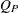, this test rejects the null hypothesis in favor of the alternative hypothesis of general association.

In addition to the asymptotic test, you can request an exact Pearson chi-square test by specifying the PCHI or CHISQ option in the EXACT statement. See the section Exact Statistics for more information.

Fortables, the Pearson chi-square is also appropriate for testing the equality of two binomial proportions. For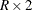and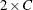tables, the Pearson chi-square tests the homogeneity of proportions. See Fienberg (1980) for details.

##### Standardized Residuals

When you specify the CROSSLIST(STDRES) option in the TABLES statement for two-way or multiway tables, PROC FREQ displays the standardized residuals in the CROSSLIST table.

The standardized residual of a crosstabulation table cell is the ratio of (frequencyexpected) to its standard error, where frequency is the table cell frequency and expected is the estimated expected cell frequency. The expected frequency is computed under the null hypothesis that the row and column variables are independent. See the section Pearson Chi-Square Test for Two-Way Tables for more information.

PROC FREQ computes the standardized residual of table cell (i, j) as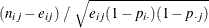whereis the observed frequency of table cell (i, j),is the expected frequency of the table cell,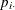is the proportion in row i (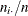), and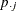is the proportion in column j (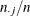). The expected frequency of table cell (i, j) is computed asUnder the null hypothesis of independence, each standardized residual has an asymptotic standard normal distribution. See section 2.4.5 of Agresti (2007) for more information.

##### Likelihood Ratio Chi-Square Test for One-Way Tables

For one-way frequency tables, the CHISQ(LRCHISQ) option in the TABLES statement provides a likelihood ratio chi-square goodness-of-fit test. By default, the likelihood ratio test is based on the null hypothesis of equal proportions in the C classes (levels) of the one-way table. If you specify null hypothesis proportions or frequencies by using the CHISQ(TESTP=) or CHISQ(TESTF=) option, respectively, the likelihood ratio test is based on the null hypothesis values that you specify.

PROC FREQ computes the one-way likelihood ratio test as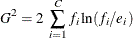whereis the observed frequency of class i, andis the expected frequency of class i under the null hypothesis.

For the null hypothesis of equal proportions, the expected frequency of each class equals the total sample size divided by the number of classes,If you provide null hypothesis frequencies by specifying the CHISQ(TESTF=) option in the TABLES statement, the expected frequencies are the TESTF= values that you specify. If you provide null hypothesis proportions by specifying the CHISQ(TESTP=) option in the TABLES statement, PROC FREQ computes the expected frequencies aswhere the proportionsare the TESTP= values that you specify.

Under the null hypothesis (of equal proportions, specified frequencies, or specified proportions), the likelihood ratio statistic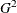has an asymptotic chi-square distribution with C–1 degrees of freedom.

In addition to the asymptotic test, you can request an exact one-way likelihood ratio chi-square test by specifying the LRCHISQ option in the EXACT statement. See the section Exact Statistics for more information.

##### Likelihood Ratio Chi-Square Test

The likelihood ratio chi-square involves the ratios between the observed and expected frequencies. The likelihood ratio chi-square statistic is computed as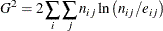whereis the observed frequency in table cell (i, j) andis the expected frequency for table cell (i, j).

When the row and column variables are independent,has an asymptotic chi-square distribution with (R–1)(C–1) degrees of freedom.

In addition to the asymptotic test, you can request an exact likelihood ratio chi-square test by specifying the LRCHI or CHISQ option in the EXACT statement. See the section Exact Statistics for more information.

The continuity-adjusted chi-square fortables is similar to the Pearson chi-square, but it is adjusted for the continuity of the chi-square distribution. The continuity-adjusted chi-square is most useful for small sample sizes. The use of the continuity adjustment is somewhat controversial; this chi-square test is more conservative (and more like Fisher’s exact test) when the sample size is small. As the sample size increases, the continuity-adjusted chi-square becomes more like the Pearson chi-square.

The continuity-adjusted chi-square statistic is computed as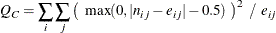Under the null hypothesis of independence,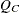has an asymptotic chi-square distribution with (R–1)(C–1) degrees of freedom.

##### Mantel-Haenszel Chi-Square Test

The Mantel-Haenszel chi-square statistic tests the alternative hypothesis that there is a linear association between the row variable and the column variable. Both variables must lie on an ordinal scale. The Mantel-Haenszel chi-square statistic is computed as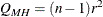where r is the Pearson correlation between the row variable and the column variable. For a description of the Pearson correlation, see the Pearson Correlation Coefficient. The Pearson correlation and thus the Mantel-Haenszel chi-square statistic use the scores that you specify in the SCORES= option in the TABLES statement. See Mantel and Haenszel (1959) and Landis, Heyman, and Koch (1978) for more information.

Under the null hypothesis of no association,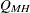has an asymptotic chi-square distribution with one degree of freedom.

In addition to the asymptotic test, you can request an exact Mantel-Haenszel chi-square test by specifying the MHCHI or CHISQ option in the EXACT statement. See the section Exact Statistics for more information.

##### Fisher’s Exact Test

Fisher’s exact test is another test of association between the row and column variables. This test assumes that the row and column totals are fixed, and then uses the hypergeometric distribution to compute probabilities of possible tables conditional on the observed row and column totals. Fisher’s exact test does not depend on any large-sample distribution assumptions, and so it is appropriate even for small sample sizes and for sparse tables.

###### 22 Tables

Fortables, PROC FREQ gives the following information for Fisher’s exact test: table probability, two-sided p-value, left-sided p-value, and right-sided p-value. The table probability equals the hypergeometric probability of the observed table, and is in fact the value of the test statistic for Fisher’s exact test.

Where p is the hypergeometric probability of a specific table with the observed row and column totals, Fisher’s exact p-values are computed by summing probabilities p over defined sets of tables,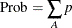The two-sided p-value is the sum of all possible table probabilities (conditional on the observed row and column totals) that are less than or equal to the observed table probability. For the two-sided p-value, the set A includes all possible tables with hypergeometric probabilities less than or equal to the probability of the observed table. A small two-sided p-value supports the alternative hypothesis of association between the row and column variables.

Fortables, one-sided p-values for Fisher’s exact test are defined in terms of the frequency of the cell in the first row and first column of the table, the (1,1) cell. Denoting the observed (1,1) cell frequency by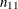, the left-sided p-value for Fisher’s exact test is the probability that the (1,1) cell frequency is less than or equal to. For the left-sided p-value, the set A includes those tables with a (1,1) cell frequency less than or equal to. A small left-sided p-value supports the alternative hypothesis that the probability of an observation being in the first cell is actually less than expected under the null hypothesis of independent row and column variables.

Similarly, for a right-sided alternative hypothesis, A is the set of tables where the frequency of the (1,1) cell is greater than or equal to that in the observed table. A small right-sided p-value supports the alternative that the probability of the first cell is actually greater than that expected under the null hypothesis.

Because the (1,1) cell frequency completely determines thetable when the marginal row and column sums are fixed, these one-sided alternatives can be stated equivalently in terms of other cell probabilities or ratios of cell probabilities. The left-sided alternative is equivalent to an odds ratio less than 1, where the odds ratio equals (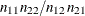). Additionally, the left-sided alternative is equivalent to the column 1 risk for row 1 being less than the column 1 risk for row 2,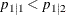. Similarly, the right-sided alternative is equivalent to the column 1 risk for row 1 being greater than the column 1 risk for row 2,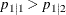. See Agresti (2007) for details.

###### RC Tables

Fisher’s exact test was extended to generaltables by Freeman and Halton (1951), and this test is also known as the Freeman-Halton test. Fortables, the two-sided p-value definition is the same as fortables. The set A contains all tables with p less than or equal to the probability of the observed table. A small p-value supports the alternative hypothesis of association between the row and column variables. Fortables, Fisher’s exact test is inherently two-sided. The alternative hypothesis is defined only in terms of general, and not linear, association. Therefore, Fisher’s exact test does not have right-sided or left-sided p-values for generaltables.

Fortables, PROC FREQ computes Fisher’s exact test by using the network algorithm of Mehta and Patel (1983), which provides a faster and more efficient solution than direct enumeration. See the section Exact Statistics for more details.

##### Phi Coefficient

The phi coefficient is a measure of association derived from the Pearson chi-square. The range of the phi coefficient is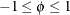fortables. For tables larger than, the range is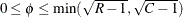(Liebetrau, 1983). The phi coefficient is computed as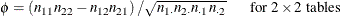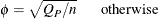##### Contingency Coefficient

The contingency coefficient is a measure of association derived from the Pearson chi-square. The range of the contingency coefficient is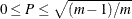, where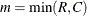(Liebetrau, 1983). The contingency coefficient is computed as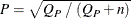Cramér’s V is a measure of association derived from the Pearson chi-square. It is designed so that the attainable upper bound is always 1. The range of Cramér’s V is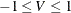fortables; for tables larger than, the range is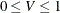. Cramér’s V is computed as